Next: 1.4 More Sets Up: 1. Notation, Undefined Concepts Previous: 1.2 Propositions   Index

# 1.3 Equality

1.34   Notation (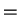.) Letand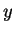be (names of) objects. I write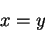to mean thatandare names for the same object. I will not make a distinction between an object and its name.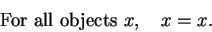(1.35)

We describe this property by saying that equality is reflexive.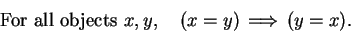(1.36)

We describe this property by saying that equality is symmetric.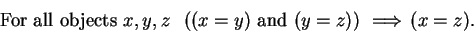(1.37)

We describe this property by saying that equality is transitive.

Letbe a proposition involving the object. Let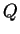be a proposition obtained by replacing any or all occurrences ofinby. Then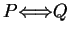. We call this property of equality the substitution property.

1.38   Examples. Suppose that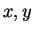are integers, and. Thenand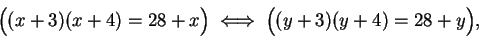and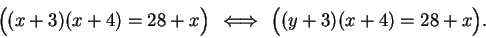We will frequently make statements likeThe justification for this is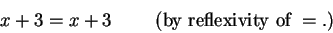Hence, if, then by the substitution property,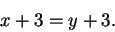1.39   Warning. Because we are using a vague notion of proposition, the substitution property of equality as stated is not precisely true. For example, although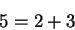(1.40)

and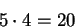(1.41)

are both true, the result of substituting the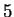in the second equation by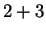yields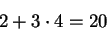which is false.

The proper conclusion that follows from (1.40) and (1.41) is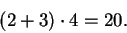(The use of parentheses is discussed in Remark 2.50.)

1.42   Notation (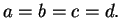) Let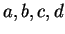be objects. We write(1.43)

as an abbreviation for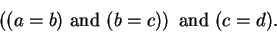If (1.43) is true, then by several applications of transitivity, we conclude that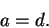Next: 1.4 More Sets Up: 1. Notation, Undefined Concepts Previous: 1.2 Propositions   Index